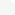# Categories# Video Training → Cool Math Guy - Algebra ICool Math Guy - Algebra I
DVDRips | MP4/AVC, ~499 kb/s | 496x372 | Duration: 21:00:09 | English: AAC, 117 kb/s (1 ch) | + PDF Guide
Size: 6.14 GB | Genre: Math

Core topics include solving linear equations and inequalities, graphing equations and inequalities with some use of the graphing calculator, exponents, polynomials, factoring, rational expressions and equations, systems of linear equations and inequalities, radical expressions and equations, and solving quadratic equations. Algebra I follows Prealgebra in the sequence of math courses and is often used as a developmental course at the college level under the name Elementary Algebra.

# Updates

«    May 2019    »
MonTueWedThuFriSatSun
12345
6789101112
13141516171819
20212223242526
2728293031

# Friend Sites

» ReleaseLog
» Gfxtorrent

Your Link Here ?
(Pagerank 4 or above)